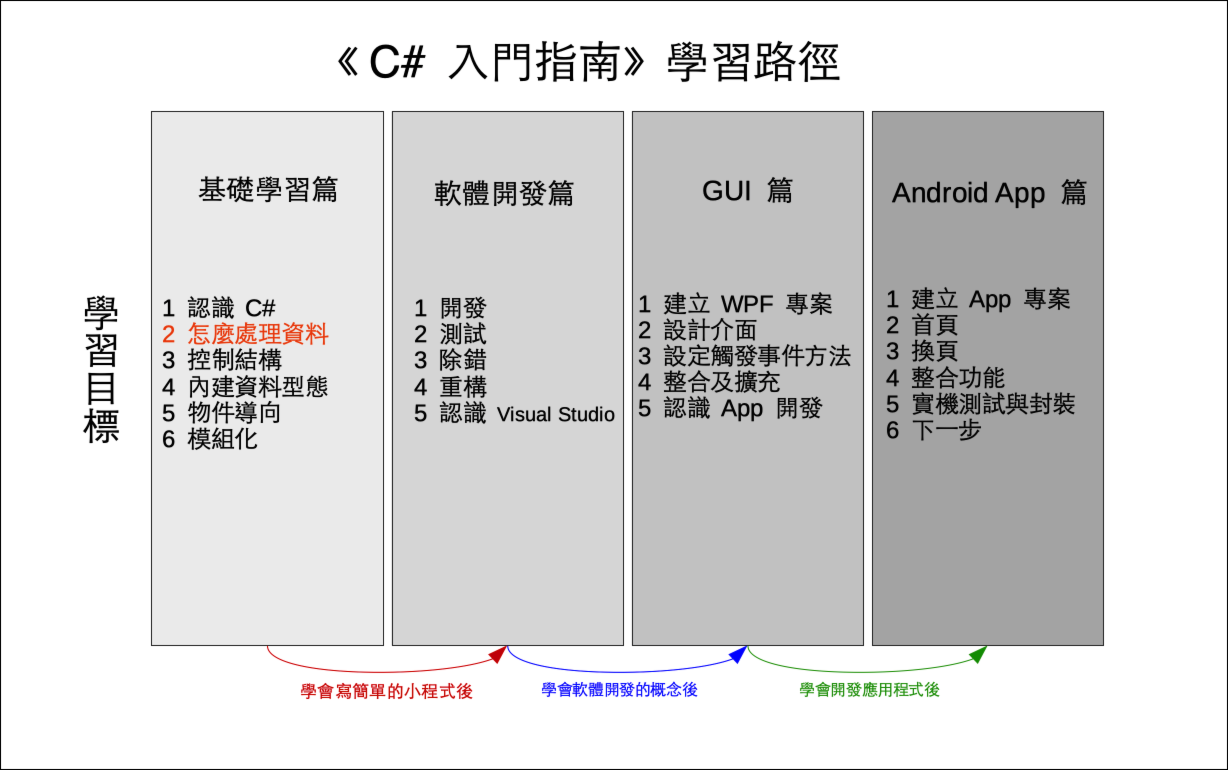# 單元 5 - 陳述與運算式

～～學習進度表～～statement;

C# 的陳述只能有以下四種

• 宣告 (declaration) 與指派 (assignment) ；
• 呼叫 (call) ；
• 遞增 (increment) 或遞減 (decrement) ；
• 建立新的物件 (object) 或使用陳述式關鍵字 (statement keyword) 。

``int a = 0;``

``Console.WriteLine();``

``````a++;
a--;``````

``new int();``

++a
--a

``````using System;

namespace ExpDemo01
{
class Program
{
static void Main(string[] args)
{
int a = -1;
int b = +a;
int c = -a;
Console.WriteLine(b);
Console.WriteLine(c);
}
}
}

//《程式語言教學誌》的範例程式
// http://kaiching.org/
// 專案：ExpDemo01
// 檔名：Program.cs
// 功能：示範 C# 的運算式
// 作者：張凱慶``````

 C:\ExpDemo01> dotnet run -1 1 C:\ExpDemo01>

&a & b
&&條件式且a && b
|a | b
||條件式或a | b
^互斥或a ^ b
!!a

! 也是單元運算子， &&&|||^ 則需要兩個運算元，例如

``````using System;

namespace ExpDemo02
{
class Program
{
static void Main(string[] args)
{
bool a = true;
bool b = false;
Console.WriteLine(a & b);
Console.WriteLine(a | b);
Console.WriteLine(a ^ b);
Console.WriteLine(!a);
}
}
}

//《程式語言教學誌》的範例程式
// http://kaiching.org/
// 專案：ExpDemo02
// 檔名：Program.cs
// 功能：示範 C# 的運算式
// 作者：張凱慶``````

 C:\ExpDemo02> dotnet run False True True False C:\ExpDemo02>

++遞增a++
--遞減a--
+a + b
-a - b
*a * b
/a / b
%取餘數a % b

``````using System;

namespace ExpDemo03
{
class Program
{
static void Main(string[] args)
{
double a = 100.0;
double b = 5.0;
a++;
Console.WriteLine(a);
a--;
Console.WriteLine(a);
Console.WriteLine(a + b);
Console.WriteLine(a - b);
Console.WriteLine(a * b);
Console.WriteLine(a / b);
Console.WriteLine(a % b);
}
}
}

//《程式語言教學誌》的範例程式
// http://kaiching.org/
// 專案：ExpDemo03
// 檔名：Program.cs
// 功能：示範 C# 的運算式
// 作者：張凱慶``````

 C:\ExpDemo03> dotnet run 101 100 105 95 500 20 0 C:\ExpDemo03>

==相等a == b
!=不相等a != b
>大於a > b
>=大於等於a >= b
<小於a < b
<=小於等於a <= b

``````using System;

namespace ExpDemo04
{
class Program
{
static void Main(string[] args)
{
int a = 11;
int b = 22;
bool c = a >= b;
Console.WriteLine(c);
bool d = a <= b;
Console.WriteLine(d);
bool e = a == b;
Console.WriteLine(e);
bool f = a != b;
Console.WriteLine(f);
}
}
}

//《程式語言教學誌》的範例程式
// http://kaiching.org/
// 專案：ExpDemo04
// 檔名：Program.cs
// 功能：示範 C# 的運算式
// 作者：張凱慶``````

 C:\ExpDemo04> dotnet run False True False True C:\ExpDemo04>

=指派a = b
+=相加同時指派a += b
-=相減同時指派a -= b
*=相乘同時指派a *= b
/=相除同時指派a /= b
%=取餘數同時指派a %= b
**=取指數同時指派a **= b

``````using System;

namespace ExpDemo05
{
class Program
{
static void Main(string[] args)
{
int a = 1;
a += 2;
a -= 2;
a *= 2;
a /= 2;
a %= 2;
Console.WriteLine(a);
}
}
}

//《程式語言教學誌》的範例程式
// http://kaiching.org/
// 專案：ExpDemo05
// 檔名：Program.cs
// 功能：示範 C# 的運算式
// 作者：張凱慶``````

 C:\ExpDemo05> dotnet run 1 C:\ExpDemo05>

``bool f = (a != b);``1. C# 參考 | Microsoft Docs - C# 運算子
2. C# 參考 | Microsoft Docs - 算術運算子
3. C# 參考 | Microsoft Docs - 布林值邏輯運算子
4. C# 參考 | Microsoft Docs - 等號比較運算子
5. C# 參考 | Microsoft Docs - 比較運算子
6. C# 參考 | Microsoft Docs - = 運算子
7. C# 參考 | Microsoft Docs - + 及 += 運算子
8. C# 參考 | Microsoft Docs - - 及 -= 運算子
9. C# 參考 | Microsoft Docs - new 運算子

1. 陳述是程式中執行的單位， C# 中的陳述要以分號結尾。
2. C# 的陳述只有四種，分別是宣告與指派、呼叫、遞增或遞減、建立新的物件或使用陳述式關鍵字。
3. 運算式由運算元與運算子組合而成。
4. 正號不會改變運算元的正負值，負號會負負得正。
5. 邏輯運算子包括且、條件式且、或、條件式或、互斥或、非。
6. 算術運算子包括遞增、遞減、加、減、乘、除、取餘數。
7. 比較運算子包括相等、不相等、大於、大於等於、小於、小於等於。
8. 等號是指派運算子，指派運算子可以和算術運算子、邏輯運算子連在一起用。
9. 小括弧具有最高的計算優先次序。

1. 邏輯運算子可以運用在整數、浮點數或字串嗎？
2. 字串為什麼可以用加法？那也可以用乘法、減法或除法嗎？
3. 為什麼測試兩個變數相等的運算子為連續兩個等號？
4. 為什麼計算的優先次序很重要？

1. 建立一個新專案 Exercise0501 ，建立兩個 int 變數 aba 設定為 2b 設定為 8 ，利用 +-*/% 計算出兩者加、減、乘、除、取餘數的結果。
2. 承上題，另建立一個新專案 Exercise0502 ，利用 ConsoleReadLine() 方法取得使用者輸入的字串，並用 Convert.ToInt32() 轉換為整數，將取得的整數指派給 ab 後，然後再計算出結果。
3. 建立一個新專案 Exercise0503 ，宣告一個字串變數 a ，並且將 a 的值設定為 "*" ，然後印出 aa + aa + a + aa + a + a + aa + a + a + a + a